Example Questions

Example Question #31 : Outcomes

What is the probability of rolling a total of 5 with a single roll of two fair 6-sided dice, each with the distinct numbers 1 through 6 on each side?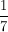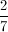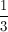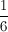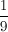Explanation: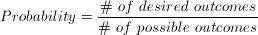When one die is rolled, there are six possible outcomes, when two dice are rolled, there are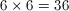possible outcomes. Getting a total value of 5 can be achieved in the following ways: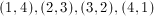So four possible ways. So the probability of rolling a total of 5 is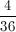, which is.

Example Question #31 : Outcomes

A fair six-sided die is thrown.

Quantity A: The probability that the die will show either an even number or a 1

Quantity B: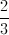Quantity B is greater

The two quantities are equal

The information cannot be determined from the information given

Quantity A is greater

The two quantities are equal

Explanation:

A die has six sides and there are 3 possible even numbers that the die could land on. Thus the probability that the die will land on an even number (2,4,6) or a 1 is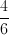, or.

Example Question #43 : Data Analysis

A six-sided die is thrown twice. What is the probability that both numbers are 5 or 6?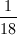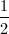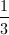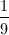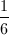Explanation:

The probability of each die rolling a 5 or 6 is. The probability of both rolls being 5 or 6 is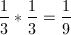.

Example Question #44 : Data Analysis

In a regular deck of cards, what is the probability that a card drawn at random will be a diamond or a face card?

Aces are not a face card.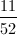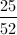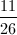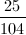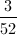Explanation:

In a regular deck, there are 13 cards of each suit, so 13 diamonds. Also, there are four cards of each rank, so the set of face cards contains 12 cards in total (4 kings, 4 queens, and 4 jacks).

If these sets of diamonds and face cards did not overlap, there would be 13 + 12 = 25 cards of interest to choose; however, three face cards are also diamonds (king of diamonds, queen of diamonds, jack of diamonds), so we need to subtract 3 from the combined set to avoid double-counting, giving a total of 22 cards of interest.

Finally, to get the probability, we divide the number of cards of interest by the total number of cards in the deck.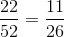Example Question #32 : Probability

There are 15 people in a room. What is the probablility that at least 3 of them were born on the same day of the week?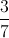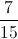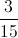Explanation:

The probability is 1 because since there are 15 people and only 7 days of the week.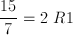This means even if each of the 7 days only had 2 people's birthday on them after checking the first 14 people the 15th and final person must also have a birthday on one of these days, so at the very least one day of the week will include at least 3 people's birthday.

Example Question #41 : Data Analysis

The probability that event A occurs is 0.22. The probability of event B occuring is 0.35. If the probability of event C occuring is 0.33, and the probability of event A or event B occuring is 0.54, then the probability of event C occuring is how many times the probability of event A and B occuring?

10

11

33

3

57

11

Explanation:

Keywords in this question are or and and. Here, the probability of event A or B is p(A) + p(B) if they are mutually exclusive. If they are not, however, the probability of A or B is p(A) + p(B) - p(A and B).

Mutually exclusive: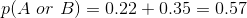Not mutually exclusive: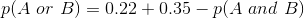Given that p(A) + p(B) = 0.57, and we are told that p(A or B) = 0.54, it implies that p(A and B) = 0.03 (and that A and B are not mutually exclusive).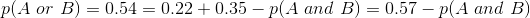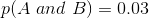Now we can solve to ratio of p(A and B) to p(C).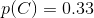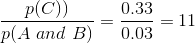Example Question #42 : Data Analysis

Two six-sided dice are rolled.

Quantity A: The probability of rolling a total of 6

Quantity B: The probability of rolling a total of 9

Quantity A is greater

The relationship cannot be determined from the information given.

Quantity B is greater

The two quantities are equal

Quantity A is greater

Explanation:

List out the the possibilities of combinations for rolling a total of 6 and the possibilities of combinations for rolling a total of 9. There are 5 possible outcomes for rolling a total of 6 and 4 possible outcomes for rolling a total of 9. There are a total of 36 possible outcomes, so the probability of rolling a 6 is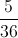and the possibility of rolling a 9 is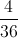Example Question #41 : Data Analysis

James has three normal six-sided dice. He rolls all three of the dice at the same time and records the three numbers that land face up. What is the probability that the dice did NOT land on three-of-a-kind?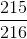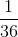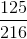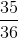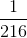Explanation:

The probability of an event not occurring is defined as 1 minus the probability of that event occurring. The probability of rolling three of a kind on every face is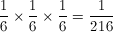.

There are six different possible three-of-a-kinds, so the probability is therefore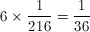.

Therefore the probability of not rolling a three of the same number is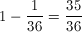.

Example Question #41 : Probability

There are seven black marbles and nine white marbles in a bag. What is the approximate probability of drawing two black marbles and then a white marble without replacement?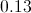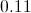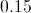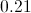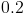Explanation:

This is a straightforward probability problem. Recall that to find the probability of related draws without replacement, we multiply the relative probabilities of each event. The first draw has a probability of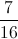, the second draw of, and the third draw of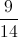.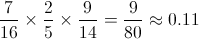Example Question #41 : Data Analysis

There is a bag of 28 red balls and a bag of 12 blue balls.

Quantity A: The total number of red and blue balls.

Quantity B: The number of blue balls that you can pair with one red ball.

The two quantities are equal

Quantity A is greater

Quantity B is greater

The relationship cannot be determined from the information given